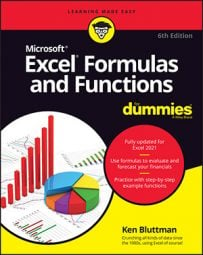##### Excel Formulas & Functions For DummiesSome Excel functions apply to specific subject areas, but others are general and apply to all needs. The following list shows an array of Excel functions used by one and all. Check here for a quickie reference to the purpose of each Excel function.
Excel Function Description
SUM Calculates the sum of a group of values
AVERAGE Calculates the mean of a group of values
COUNT Counts the number of cells in a range that contains numbers
INT Removes the decimal portion of a number, leaving just the integer portion
ROUND Rounds a number to a specified number of decimal places or digit positions
IF Tests for a true or false condition and then returns one value or another
NOW Returns the system date and time
TODAY Returns the system date, without the time
SUMIF Calculates a sum from a group of values, but just of values that are included because a condition is met
COUNTIF Counts the number of cells in a range that match a criteria